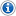## Encryption/DecryptionYou are viewing a static copy of the old 2DBoy forum, which closed in 2010. It is preserved here for historical interest, but it is not possible to reply to topics. For more recent discussion about World of Goo, visit our new forum.
 Encryption/Decryption Scaevolus 10/27/2008 - 21:26 World of Goo uses AES in CBC mode with a 192 bit key, 0D0607070C01080506090904060D030F03060E010E02070B, to encrypt files ending in .bin, along with some other files, such as user profiles. This was discovered by a user on another forum I read, who goes by the name "gibbed".Here is a simple Python program that will encrypt and decrypt files, and strip or add padding as appropriate. It uses a heuristic to detect whether a file is encrypted, so it might attempt to decrypt non-encrypted files with characters outside of the normal ASCII range, causing an error.`
#!/usr/bin/env python
import sys
from Crypto.Cipher import AES # http://www.dlitz.net/software/pycrypto/

key = "\x0D\x06\x07\x07\x0C\x01\x08\x05\x06\x09\x09\x04\x06\x0D\x03\x0F"\
"\x03\x06\x0E\x01\x0E\x02\x07\x0B"
aes = AES.new(key, AES.MODE_CBC)

if len(sys.argv) > 1:
else:

if not all(ord(c) < 128 for c in text[:100]): #check for encryption
plain = aes.decrypt(text)
plain = plain[:plain.rfind('\xfd\xfd\xfd\xfd')]
sys.stdout.write(plain)
else:
text += "\xfd"*4 + "\x00"*(16-(len(text)+4)%16)
sys.stdout.write(aes.encrypt(text))
`Here is a plaintext dump of all such encrypted files: http://cyanyde.com/~scaevolus/WorldOfGoo/ (if an admin feels that hosting this infringes on your copyright, please tell me and I will remove it) Re: Encryption/Decryption ltworek 10/27/2008 - 22:36 Wow a mods forum, this is awesome thanks guys.Anyway, heres a link to the gui app i wrote, only does one file at a time. http://www.mediafire.com/?12yfwxqjdh1Here's another python script written by SoulTaker`#!/usr/bin/env python

# Uses the Python Cryptography Toolkit
# for Windows dummies: http://www.voidspace.org.uk/python/modules.shtml#pycrypto

from Crypto.Cipher import AES
import sys
import os

# AES encryption key used (192 bits)
key = "\x0D\x06\x07\x07\x0C\x01\x08\x05\x06\x09\x09\x04\x06\x0D\x03\x0F\x03\x06\x0E\x01\x0E\x02\x07\x0B"

def decrypt(input):
aes = AES.new(key, AES.MODE_CBC)
output = aes.decrypt(input)
eof = output.find("\xfd")
if eof >= 0: output = output[:eof]
return output

def encrypt(input):
input += "\xfd\xfd"
aes = AES.new(key, AES.MODE_CBC)
return aes.encrypt(input)

if len(sys.argv) <> 2 or sys.argv not in ('-e','-d'):
print 'Usage: goocrypt -d  (decrypt files)'
print '      goocrypt -e  (encrypt files)'
sys.exit(1)
if sys.argv == '-d': what, ext_in, ext_out = 'decrypting', '.bin', '.txt'
if sys.argv == '-e': what, ext_in, ext_out = 'encrypting', '.txt', '.bin'

found = 0
for dirname in [ 'properties', 'res' ]:
for (path, dirs, files) in os.walk(dirname):
for name in files:
if name.endswith(ext_in):
found += 1
path_in  = os.path.join(path, name)
path_out = os.path.join(path, name[:-len(ext_in)]) + ext_out
print "%s %s\n => %s"% (what, path_in, path_out)
if what == 'decrypting': output = decrypt(input)
if what == 'encrypting': output = encrypt(input)
file(path_out, "wb").write(output)

print 'No files found! (Did you run from the World Of Goo directory?)'
else:
print found, 'files processed'`And then a third sent to me via pm by zark92`from __future__ import with_statement
import sys
from Crypto.Cipher import AES
import optparse

def make_aes_cipher():
"""Returns a new cipher object initialized with the correct key
that can be used to encrypt/decrypt files."""
# AES 192 bits keys used to encrypt/decrypt data
key = '0D0607070C01080506090904060D030F03060E010E02070B'

def key_as_binary( key ):
"""Converts the specified hexadecimal string into a byte string."""
assert len(key) % 2 == 0
binary_key = []
for index in xrange(0,len(key),2):
binary_key.append( chr(int(key[index:index+2],16)) )
return ''.join( binary_key )

binary_key = key_as_binary( key )
cipher = AES.new(binary_key, AES.MODE_CBC)
return cipher

def encrypt_file( input_path, output_path ):
"""Encrypt XML file input_path into .bin file output_path using AES algorithm."""
cipher = make_aes_cipher()
with file( input_path, 'rb' ) as f:
# adds filler so that input data length is a multiple of 16
filler = '\xfd\xfd\xfd\xfd' + '\0' * 12
filler_size = 16 - len(xml_data) % 16
xml_data += filler[0:filler_size]
# encrypt the data
encrypted_data = cipher.encrypt( xml_data )
with file( output_path, 'wb' ) as fout:
fout.write( encrypted_data )
print 'Encrypted "%s" into "%s"' % (input_path, output_path)
return True

def decrypt_file( input_path, output_path ):
"""Decrypt a .bin file input_path into .xml file output_path using AES algorithm."""
cipher = make_aes_cipher()
with file( input_path, 'rb' ) as f:
xml_data = cipher.decrypt( crypted_data )
with file( output_path, 'wb' ) as fout:
# clean-up the data. Usually has '\xfd\xfd\xfd\xfd\0' at the
# end, but this may be truncated to first '\xfd' if
# size is nearly a multiple of 16, though there will
# always be at least one '\xfd'.
zero_index = xml_data.find( '\0' )
if zero_index != -1:
xml_data = xml_data[:zero_index]
fd_index = xml_data.find( '\xfd' )
if fd_index != -1:
xml_data = xml_data[:fd_index]
fout.write( xml_data )
print 'Decrypted "%s" into "%s"' % (input_path, output_path)
return True

def main():
parser = optparse.OptionParser( """
1. %prog --decrypt encrypted-path decrypted-path
2. %prog --encrypt decrypted-path encrypted-path

Usage 1 will decrypt the file specified by 'encrypted-path' into the
file 'decrypted-path' (the file is overwriten if it exist').

Usage 2 will encrypt the file specified by 'decrypted-path' into the
file 'encrypted-path' (the file is overwriten if it exist').

Typically, encrypted-path file should have an extension .bin, and
decrypted-path file should have an extension .xml.
""" )
parser.add_option( '-d', '--decrypt', dest='decrypt', action="store_true", default = False,
help = 'Decrypt the input path into the output path' )
parser.add_option( '-e', '--encrypt', dest='encrypt', action="store_true", default = False,
help = 'Encrypt the input path into the output path' )
(options, args) = parser.parse_args()
if len(args) != 2:
parser.error( 'You must specify the input and ouput path' )
if args == args:
parser.error( 'Input path must be different from output path' )

if options.decrypt:
return decrypt_file( args, args )
elif options.encrypt:
return encrypt_file( args, args )
else:
parser.error( 'You must specify either --decrypt or --encrypt' )

if __name__ == '__main__':
succeed = main()
if not succeed:
print 'Failed'
sys.exit( 2 )